DOTA Data Analysis¶

Introduction¶

For our final project we analyzed the video game Defense of the Ancients 2(DOTA). For a full explanation of the game and further resources, check out http://dota2.gamepedia.com/Dota_2. DOTA is game played by ten players, five per team, who compete in matches that generally last forty-five minutes in length. Before each match, players choose a unique hero without replacement from a pool of 113 heroes, 111 of which are in the dataset, which is missing the heroes corresponding to the numbers 24 and 108. Throughout the match, players earns gold and experience which help them level up their character. Gold and experience are reset after each match.

We analyzed the data from two CSV files we received from a company called Feedless, a company creating an AI coaching bot for DOTA. The 2 CSV files are titled MatchOverview and MatchDetail.

MatchOverview contains 23875 rows, with each row corresponding to a unique match. The data frame contains 12 columns, grouped by the match id.

• The first column identifies the match, usually a large number like 2503037971.

• The next ten columns contain the heroes picked in the match; columns one through five represent team zero, columns six through ten represent team one. The columns can contain any integer 1-113 inclusive, skipping 24 and 108, which were heroes not yet released to the public.

• The final column is a boolean variable representing the winning team; 0 if team zero won and 1 if team one won.

MatchDetail contains 202319 rows and 23 columns containing data on the experience and gold for a player at 5 minute intervals up to 45 minutes. For example e_5 represents experience of a player at the 5 minute mark and g_5 represents gold of a player at the 5 minute mark.

• The first 3 columns identify the player, character and match id

• The next 10 columns detail the experience values at 5 minute intervals, from 0 minutes to 45 minutes inclusive.

• The final 10 columns detail the gold values at 5 minute intervals, from 0 minutes to 45 minutes inclusive.

Goal¶

Through our analysis, we hoped to identify if a hero would win a match based on their gold and experience throughout the match.

Data Clean¶

We began our analysis by loading the two datasets and reading over the data types and some of the observations.

In :
import pandas as pd
import numpy as np
from collections import Counter
import matplotlib.pyplot as plt
%matplotlib inline
from ggplot import *
import sklearn
In :
return overview,detail

print overview.dtypes
print "---------"
print detail.dtypes

print "---------"

match_id         int64
hero_1           int64
hero_2         float64
hero_3         float64
hero_4         float64
hero_5         float64
hero_6         float64
hero_7         float64
hero_8         float64
hero_9         float64
hero_10        float64
first_5_won     object
dtype: object
---------
match_id       int64
hero_id      float64
player_id    float64
e_0          float64
e_5          float64
e_10         float64
e_15         float64
e_20         float64
e_25         float64
e_30         float64
e_35         float64
e_40         float64
e_45         float64
g_0          float64
g_5          float64
g_10         float64
g_15         float64
g_20         float64
g_25         float64
g_30         float64
g_35         float64
g_40         float64
g_45         float64
dtype: object
match_id  hero_1  hero_2  hero_3  hero_4  hero_5  hero_6  hero_7  hero_8  \
0  2488245920      34    83.0    29.0   102.0    12.0    88.0   107.0    10.0
1  2488233366     102    63.0    34.0   100.0    60.0     1.0    38.0    48.0
2  2488231318       7   112.0     8.0    64.0    71.0     1.0    11.0    41.0
3  2488216163      74   102.0     9.0    29.0    14.0   105.0    86.0    65.0
4  2488215113      51    68.0    79.0    15.0    10.0    85.0    28.0    91.0

hero_9  hero_10 first_5_won
0    70.0     53.0        True
1    93.0     33.0       False
2   102.0     92.0        True
3    55.0    111.0        True
4   102.0     89.0        True
---------
match_id  hero_id  player_id  e_0     e_5    e_10    e_15    e_20  \
0  2488125718    102.0        0.0  0.0  1125.0  2598.0  3898.0  7084.0
1  2488125718     42.0        1.0  0.0   990.0  2414.0  4234.0  6323.0
2  2488125718     46.0        2.0  0.0  1389.0  3756.0  6280.0  8066.0
3  2488125718      7.0        3.0  0.0  1021.0  3138.0  4505.0  5962.0
4  2488125718      8.0        4.0  0.0   717.0  2204.0  3684.0  6036.0

e_25     e_30   ...     g_0     g_5    g_10    g_15    g_20    g_25  \
0  10870.0  12375.0   ...     1.0  1729.0  2981.0  4582.0  6134.0  9139.0
1  11620.0  14179.0   ...     1.0  1402.0  2756.0  4433.0  6193.0  9140.0
2  10638.0  12636.0   ...     1.0  1252.0  2883.0  4573.0  5978.0  8586.0
3   9288.0  10616.0   ...     1.0  1156.0  2758.0  3987.0  5043.0  8220.0
4   9353.0  11611.0   ...     1.0   711.0  1577.0  3186.0  5100.0  7830.0

g_30     g_35     g_40     g_45
0  10369.0  13031.0  16101.0  20492.0
1  10944.0  13240.0  16986.0  21189.0
2  10030.0  11675.0  15951.0  22944.0
3   9274.0  12389.0  15420.0  20381.0
4   9575.0  13526.0  17632.0  23432.0

[5 rows x 23 columns]

To better analyze the data, we merged the two data frames into one data frame aptly named df. Using pandas's merge we were able to merge the two data sets on match_id and remove all match id's that did not have a corollary in the other data set. Our new data set is grouped by match id, player id and character id. Unfortunately, each row had an extra column, as both data frames had the player id of the row.

In :
df = overview.merge(detail,on="match_id")
print "Unique match id's:", len(df)
print "df.dtypes:", df.dtypes
print "----------"
print df.tail(5)
print len(df['match_id'].unique())
Unique match id's: 202319
df.dtypes: match_id         int64
hero_1           int64
hero_2         float64
hero_3         float64
hero_4         float64
hero_5         float64
hero_6         float64
hero_7         float64
hero_8         float64
hero_9         float64
hero_10        float64
first_5_won     object
hero_id        float64
player_id      float64
e_0            float64
e_5            float64
e_10           float64
e_15           float64
e_20           float64
e_25           float64
e_30           float64
e_35           float64
e_40           float64
e_45           float64
g_0            float64
g_5            float64
g_10           float64
g_15           float64
g_20           float64
g_25           float64
g_30           float64
g_35           float64
g_40           float64
g_45           float64
dtype: object
----------
match_id  hero_1  hero_2  hero_3  hero_4  hero_5  hero_6  hero_7  \
202314  2502981287     104    83.0    88.0    53.0    15.0    39.0    31.0
202315  2502981287     104    83.0    88.0    53.0    15.0    39.0    31.0
202316  2502981287     104    83.0    88.0    53.0    15.0    39.0    31.0
202317  2502981287     104    83.0    88.0    53.0    15.0    39.0    31.0
202318  2502981287     104    83.0    88.0    53.0    15.0    39.0    31.0

hero_8  hero_9   ...     g_0     g_5    g_10    g_15    g_20     g_25  \
202314    93.0    18.0   ...     1.0  1155.0  3022.0  4449.0  6337.0  10910.0
202315    93.0    18.0   ...     1.0   538.0  1249.0  2669.0  4042.0   4723.0
202316    93.0    18.0   ...     1.0  1457.0  3094.0  4428.0  6319.0   8297.0
202317    93.0    18.0   ...     1.0   929.0  1741.0  3321.0  4522.0   5573.0
202318    93.0    18.0   ...     1.0   622.0  1206.0  3239.0  4479.0   7215.0

g_30     g_35     g_40     g_45
202314  14093.0  18990.0  22260.0  25535.0
202315   6501.0   9251.0  10519.0  12400.0
202316  10199.0  13878.0  16612.0  19075.0
202317   6962.0   9454.0  12149.0  13461.0
202318  11041.0  14100.0  16437.0  20394.0

[5 rows x 34 columns]
20232

After merging the data frames, we cleaned the data by:

• Tallying how many wins each team had. By splitting our data set into training and testing data, we preserved the ratio of wins to losses for team. This avoided the rare case where our training set is entirely one team winning, which would obviously ruin predictions.
• Setting hero_id and player_id to strings, so they would be interpreted as factors and not as quantatative variables.
• Reset experience and gold columns greater than match time to the last non-zero time amount. This removed zeros that could invalidate our correlations and regressions.
• Changed player_id to team_id, a binary variable representing team, as we were interested in team performances and not individual performances.
In :
def clean_data(tempdf):
newdf = tempdf
newdf = newdf.assign(team = newdf.apply(lambda x: 0 if x.player_id < 5 else 1,axis=1))
newdf[['hero_1','hero_2','hero_3','hero_4','hero_5','hero_6','hero_7','hero_8','hero_9','hero_10','hero_id']] = newdf[['hero_1','hero_2','hero_3','hero_4','hero_5','hero_6','hero_7','hero_8','hero_9','hero_10','hero_id']].astype(str)
#on this line we can check to make sure every game has a full roster of players (having 10 players).
#When we run the above line we see that there is at least 1 game without a full roster!
C = Counter(newdf['match_id'])
less = [x for x in C.keys() if C[x] < 10]

#We run in to an issue where each player_id does not occur the same amount of times.
newdf = newdf[newdf['match_id'] != less]

#Once we emilinate these rows we have only full game data
#Now we want to change all values of 0 after the match ends into the last non-zero value
newdf = newdf.apply(lambda x: set_zero(x),axis=1)

#We want to count the number of games each team wins
counts = newdf['first_5_won'].value_counts()

newdf.columns = newdf.columns.str.replace('g_5','g_05')
newdf.columns = newdf.columns.str.replace('e_5','e_05')
newdf = newdf.assign(won = newdf.apply(lambda x: 1 if (x.first_5_won and x.team == 0) or (x.first_5_won == False and x.team == 1) else 0, axis=1))
newdf.drop('first_5_won', axis=1, inplace=True)
newdf.to_csv("output.csv")
return newdf,counts

#This is the function that changes all of the 0's at the end of every match to the last value that was non-zero for gold/experience
def set_zero(row):
value = 5
while value <= 40:
e1 = 'e_' + str(value+5)
e2 = 'e_' + str(value)
g1 = 'g_' + str(value+5)
g2 = 'g_' + str(value)
if row[e1] == 0:
row[e1] = row[e2]
row[g1] = row[g2]
value += 5
return row

df = overview.merge(detail,on="match_id")
df,counts = clean_data(df)
print df.dtypes
match_id       int64
hero_1        object
hero_2        object
hero_3        object
hero_4        object
hero_5        object
hero_6        object
hero_7        object
hero_8        object
hero_9        object
hero_10       object
hero_id       object
player_id    float64
e_0          float64
e_05         float64
e_10         float64
e_15         float64
e_20         float64
e_25         float64
e_30         float64
e_35         float64
e_40         float64
e_45         float64
g_0          float64
g_05         float64
g_10         float64
g_15         float64
g_20         float64
g_25         float64
g_30         float64
g_35         float64
g_40         float64
g_45         float64
team           int64
won            int64
dtype: object

Exploratory Data Analysis¶

Prior to modeling, we plotted a few of the variables from our dataset. The resulting plots and our analysis is below.

In :
hero_bar = ggplot(df, aes(x = 'hero_id'))

hero_bar = hero_bar + \
xlab("Hero ID") + \
ylab("Frequency") + \
ggtitle("Frequency of Hero ID")

hero_bar + geom_bar() + theme(x_axis_text=element_text(angle=90, size = 5))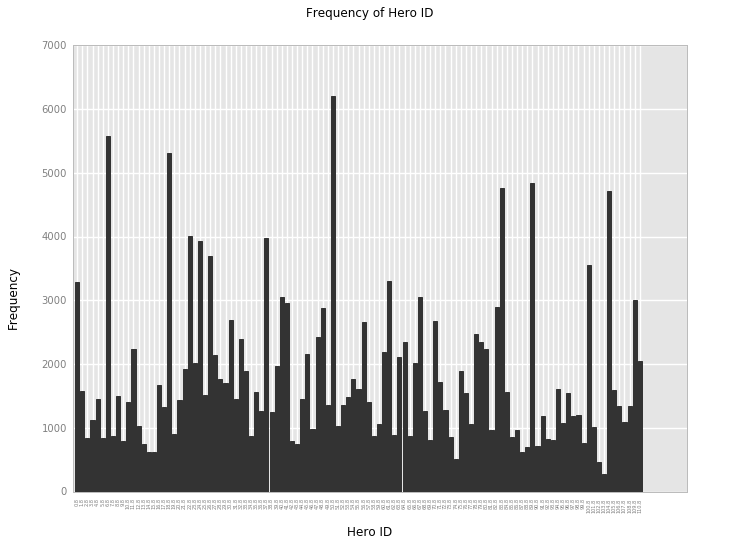Out:
<ggplot: (56931069)>

Above, we plotted the distribution of characters used in out data set. Since the characters are all distinct and not numerical, we used a barchart instead of a histogram to display the data. The most used character was character 44 (phantom Assassin http://dota2.gamepedia.com/Phantom_Assassin) and the least used character was character 92 (visage http://dota2.gamepedia.com/Visage). Overall, characters were used on average 1822 times with standard deviation of 1168. While there were deviations in which characters were used, we are uncertain whether those deviations would result in significant differences in winnings. Therefore, we used hero_id as a predictor.

We then examined gold and experience over a single match to understand relationships between gold and experience per team.

In :
match = df[df['match_id'] == 2502981287]

def colSum(df, choice, team):
c_sum = sum(df.loc[df['team'] == team, choice])
return c_sum

def timeRow(df, time):
if time == 5:
g = "g_05"
e = "e_05"
else:
g = "g_" + str(time)
e = "e_" + str(time)
index = [time]
row_d = {
"time" : time,
"gold_0" : colSum(df, g, 0),
"exp_0" : colSum(df, e, 0),
"gold_1" : colSum(df, g, 1),
"exp_1" : colSum(df, e, 1)
}
row = pd.DataFrame(data=row_d, index=index)
return row

g_e = timeRow(match, 0)
for i in range(5, 50, 5):
g_e = g_e.append(timeRow(match, i), ignore_index = True)

g_e = g_e.reset_index()
g_e = g_e.drop('index', 1)

g_e_melt = pd.melt(g_e, id_vars = "time")

g_e_point = ggplot(g_e_melt, aes(x = "time", y = "value", color = "variable"))

g_e_point = g_e_point + \
xlab("Time") + \
ylab("Value") + \
ggtitle("Experience and Gold over Time")

g_e_point + geom_line()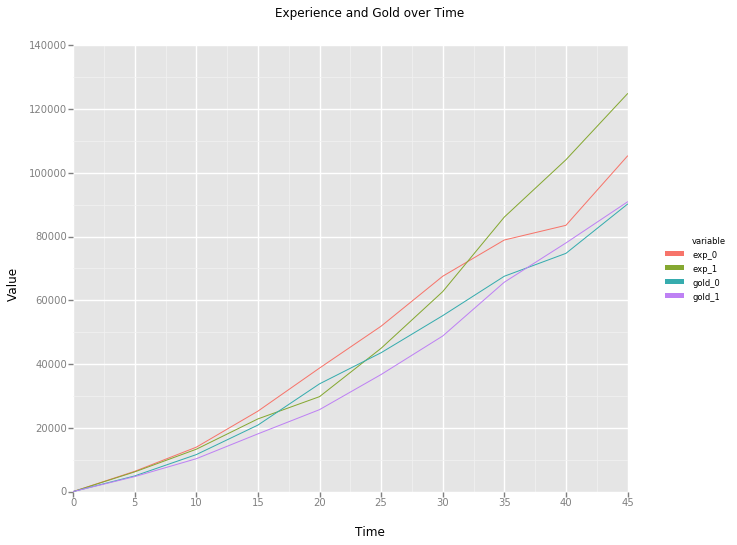Out:
<ggplot: (29951127)>

Above, we plotted the two teams' experience and gold throughout one match, 2502981287. The red and green lines represent experience, while the blue and purple lines represent gold. In the example above, team 1 (the second team) won the match. Looking at the differences between experience, there was a shift in winning between the 30th and 35th minute. The change in gold follows between the 35th and 40th minutes. I (Isaac) posit that based on this plot and other matches we have plotted, experience and gold prior to 20 minutes before the end of the match will not be significant predictors and will not add much to our model.

The plot below shows the same information, but with team 1's experience and gold subtracted from team 0's. The peak of the experience line is at 20 minutes, followed by an immediate downwards trend and a more substantial downwards trend between the 30th and 40th minutes. The significant negative difference between the experiences is similar information to the swap in experience curves above. The gold curve tracks the experience curve for most of the match and only begins to differ greatly from experience towards the 35th minute. We saw this same feature on the plot above with the convergence towards zero shown above as well.

The gold and experience graphs often go in favor of one team and then the other, not necessarily always with 1 team ahead. The issue that arises from this is that you cannot use the gold and experience at a specific time (say g_25 and e_25) to make predictions because often the graph will be rather up and down. I (Adin) posit that we are concerned with the values throughout the match since winning the game will result in large spikes of gold and experience, so no speicifc time in the game will be an accurate predictor.

In :
g_e_diff = g_e
g_e_diff['exp_diff'] = g_e['exp_0'] - g_e['exp_1']
g_e_diff['gold_diff'] = g_e['gold_0'] - g_e['gold_1']

g_e_diff = g_e_diff.drop('exp_0', 1)
g_e_diff = g_e_diff.drop('exp_1', 1)
g_e_diff = g_e_diff.drop('gold_0', 1)
g_e_diff = g_e_diff.drop('gold_1', 1)

g_e_diff_melt = pd.melt(g_e_diff, id_vars = "time")

g_e_diff_point = ggplot(g_e_diff_melt, aes(x = "time", y = "value", color = "variable"))

g_e_diff_point = g_e_diff_point + \
xlab("Time") + \
ylab("Value") + \
ggtitle("Experience and Gold over Time")

g_e_diff_point + geom_line()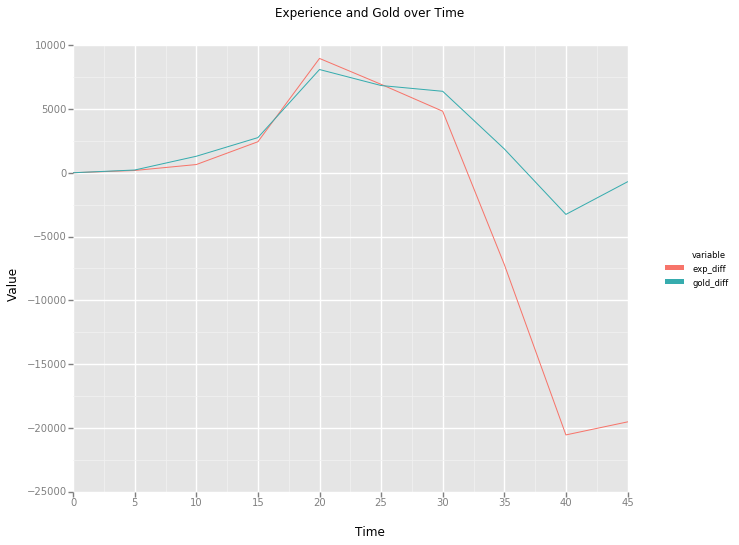Out:
<ggplot: (53407618)>
In :
g_melt = pd.melt(df[["g_0", "g_05", "g_10", "g_15", "g_20", "g_25", "g_30", "g_35", "g_40", "g_45"]])

g_hist = ggplot(g_melt, aes(x = "value"))

g_hist = g_hist + \
xlab("Gold") + \
ylab("Frequency") + \
ggtitle("Distribution of Gold\nFaceted by Time")

g_hist + geom_histogram() + facet_grid("variable")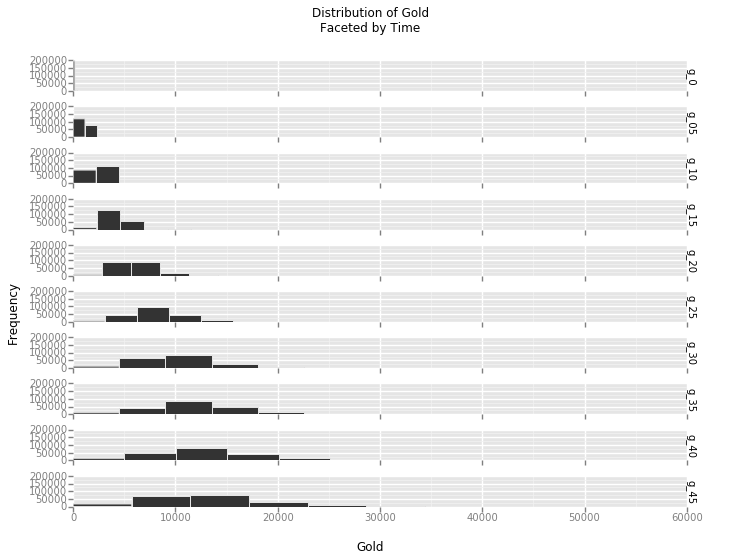Out:
<ggplot: (74596559)>

The faceted plots above show the distribution of gold at times 0 through 45 minutes. Distributions grow wider with increasing time, as certain players play well rewarding them with more gold, while others play poorly, keeping their gold totals closer to 0.

• On average, the rate of increase in gold value increases as the game progresses. As players accumulate more gold, they collect more items, which in turn allows for better killing ability and subsequently more gold. In DOTA, the bounty for a kill is based on the net worth of the player who dies, as well as the difference in gold between the two teams.

• The later in the game, the higher the net worth of the dying player. As a result, deaths closer to the end of the game cause a larger shift in net worth for the two teams.

In :
e_melt = pd.melt(df[["e_0", "e_05", "e_10", "e_15", "e_20", "e_25", "e_30", "e_35", "e_40", "e_45"]])

e_hist = ggplot(e_melt, aes(x = "value"))

e_hist = e_hist + \
xlab("Experience") + \
ylab("Frequency") + \
ggtitle("Distribution of Experience\nFaceted by Time")

e_hist + geom_histogram() + facet_grid("variable")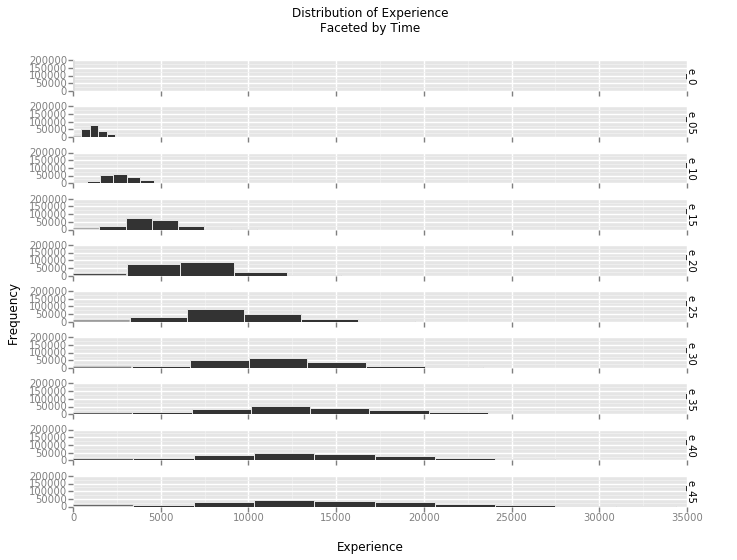Out:
<ggplot: (74138014)>

The faceted plot above shows experience plotted against the different times in the dataset. Similar to the gold dataset, as time increases, the distributions of experience widen.

• This plot shows us that there is a wider variance of gold and experience as the game progresses. Since the variance is larger, the later times may be more influencial as predictors since the grpahs for the teams may vary by more.

• Similar to above, a dying player rewards the other team with more experience the higher their own experience is, so there tends to be larger shifts later in the game.

Elo-Ratings¶

After our adventure into plotting and EDA, we explored Elo ratings of the heroes (further explanation for Elo ratings can be found at this link: http://www.herbrich.me/papers/trueskill.pdf). Using a k of 10, our ratings ranged from 1168 to 818 with a standard deviation of 67. We chose a k of 10 after testing different values of k between 5 and 25. We liked 10 because it had a very round distribution with both the maximum and minimum values close to two standard deviations from the mean.

In :
return int(round(val+10**(-len(str(val))-1)))

heroes = {str(float(i)): for i in range(1,114)}

def elo(group,heroes,k=10):
groups = group.groupby(['team'])
for name,group in groups:
if group['team'].iloc == 0:
team0Score = 0
team0Heroes = group['hero_id']
for hero in team0Heroes:
team0Score += heroes[hero][-1]

if group['won'].iloc == 1:
team0Won = 1
team1Won = 0
else:
team0Won = 0
team1Won = 1
else:
team1Score = 0
team1Heroes = group['hero_id']
for hero in team1Heroes:
team1Score += heroes[hero][-1]

for hero in team0Heroes:
for hero in team1Heroes:
return heroes

grouped = df.groupby(['match_id'])
ints = 0
for name,group in grouped:
heroes = elo(group,heroes,10)
In :
number = {str(float(i)):0 for i in range(1,114)}

def MakeElo(x,number,heroes):
elo = heroes[x.hero_id][number[x.hero_id]]
number[x.hero_id] = number[x.hero_id] + 1
return elo

df = df.assign(Elo = df.apply(lambda x: MakeElo(x,number,heroes),axis=1))
In :
df54 = df[df['hero_id'] == "54.0"]

dfElo = pd.melt(df54, value_vars = ['Elo'], id_vars = ['match_id'])

#dfElo["hero_id"] = df["hero_id"].astype(float)

times = list(range(1, len(dfElo) + 1))

dfElo['time'] = times

elo_point = ggplot(dfElo, aes(x = "time", y = "value"))

elo_point = elo_point + \
xlab("Time") + \
ylab("Value") + \
ggtitle("Elo Rating vs. Time")

elo_point + geom_line()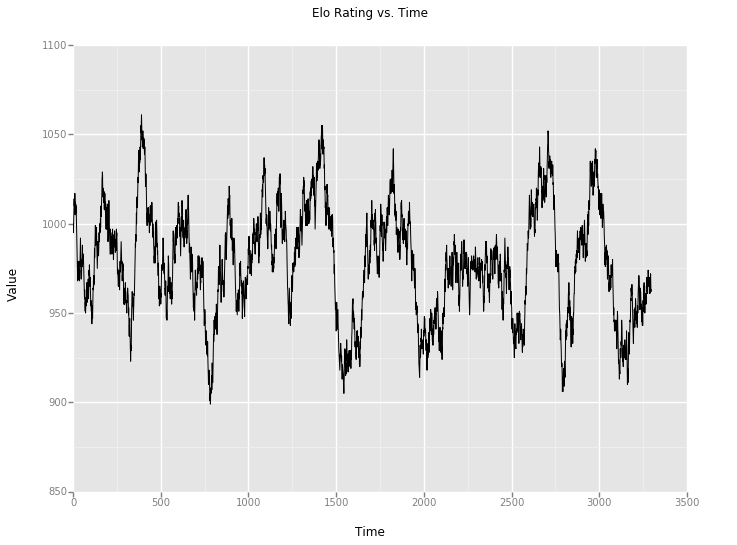Out:
<ggplot: (23875521)>

Above, we plotted the Elo rating for hero 54. The x-axis represents the matches over time, while the y-axis is the Elo rating. With a k of 10, there is variation in the Elo Ratings throughout the data set, however the final rating is close to the initial rating of 1000.

Modeling¶

After adding the Elo ratings to our data set, we began our modeling. We split the data into train and test data and tested two theories across multiple different algorithms and two theories mathematically. Our four theories were:

• Experience Theory: A team's summed experience at time 20 as the predictor for victory. Our EDA showed that the greatest disparities between teams began around 20 minutes. Therefore, we theorize that victory can be predicted from the team with more experience at time 20.

• Elo Theory: A team's summed Elo ratings as the predictor for victory. Our foray into Elo ratings showed that there was fluctuation between ratings between matches, but, generally, the preferred heroes were the ones with higher Elo ratings.

• Full Theory: Use all the variables in our data set and a variety of machine learning algorithms to find the best model.

• Twenty Theory: A combination of theories 1 and 3. All the variables except for experience and gold before time 20 will be used in the same machine learning algorithms with the same test data as the Full Theory.

I (Isaac) assumed that since the Twenty Theory was similar enough to the Full Theory and that experience at time 20 and afterwards was most important, the Full and Twenty Theories would have little variation in the accuracies.

In :
df = df.iloc[np.random.permutation(len(df))]

key = np.random.rand(len(df)) < 0.8

f_train_X = df[key].drop(["match_id", 'won'],1)
f_train_y = df[key]['won']

f_test_X = df[~key].drop(["match_id", 'won'],1)
f_test_y = df[~key]['won']

g_train_X = df[key].drop(["match_id", "g_0", "g_05", "g_10", "g_15", "e_0", "e_05", "e_10", "e_15", "won"],1)
g_train_y = df[key]['won']

g_test_X = df[~key].drop(["match_id", "g_0", "g_05", "g_10", "g_15", "e_0", "e_05", "e_10", "e_15", "won"],1)
g_test_y = df[~key]['won']
In :
def guessExp(group):
sum0 = np.sum(group[group['team'] == 0]['e_20'])
sum1 = np.sum(group[group['team'] == 1]['e_20'])
if sum0 > sum1:
group = group.assign(Exp_predicted = group.apply(lambda x: 1 if x.team == 0 else 0,axis=1))
else:
group = group.assign(Exp_predicted = group.apply(lambda x: 1 if x.team == 1 else 0,axis=1))
return group

groups_exp = df.groupby(['match_id'])
i = 0
for name,group in groups_exp:
if i == 0:
data = guessExp(group)
else:
data = data.append(guessExp(group))
i += 1
continue
In :
print "Experience Accuracy: ", np.sum(data['won'] == data['Exp_predicted'])/float(len(data))
Experience Accuracy: 0.850032128911

We began our model testing by testing the Experience Theory. We summed the rows where the team with more experience at time 20 won divided by the entire data set. This theory correctly predicted the winners of a match 85.00% of the time. Meaning that, in 85% of matches, the winner could be predicted half way through the match. This theory also informed us on the Full and Twenty theories. If by summation alone a match could be accurately predicted 85% of the time, surely by using a model with some of the later times, we could have even better predictions.

In [ ]:
def guessElo(group):
sum0 = np.sum(group[group['team'] == 0]['ELO'])
sum1 = np.sum(group[group['team'] == 1]['ELO'])
if sum0 > sum1:
group = group.assign(ELO_predicted = group.apply(lambda x: 1 if x.team == 0 else 0,axis=1))
else:
group = group.assign(ELO_predicted = group.apply(lambda x: 1 if x.team == 1 else 0,axis=1))
return group

groups = df.groupby(['match_id'])
i = 0
for name,group in groups:
if i == 0:
data = guessElo(group)
else:
data = data.append(guessElo(group))
i += 1
continue
In :
print "Elo Accuracy: ", np.sum(data['won'] == data['ELO_predicted'])/float(len(data))
0.661361277248

Our Elo Theory worked on the same principles as our Experience Theory, but only produced an accuracy of 66.13%. We believe the lower accuracy of this theory is due to the instability of Elo ratings across players and time and that the Elo ratings may not have been as stable as we theorized above. Since we cannot control for different players using different heroes, the Elo ratings had more fluctuations and instability across our data set than we would have liked.

While testing our first two theories required only summation, our other two theories required the use of machine learning algorithms. The first algorithm we tested with our theories was a randomForest. We chose to use a randomForest, because we theorized the randomForest would classify the data into certain win conditions which would best display the best predictors for victory. The randomForest produced the best accuracy of any of the algorithms we tested, with an accuracy of 86.42% for the Full theory and an accuracy of 86.45% for the Twenty theory.

In :
from sklearn.ensemble import RandomForestClassifier

f_rf_model = RandomForestClassifier(n_estimators=50, max_depth=None, min_samples_split=2, random_state=0)
f_rf_model.fit(f_train_X,f_train_y)

g_rf_model = RandomForestClassifier(n_estimators=50, max_depth=None, min_samples_split=2, random_state=0)
g_rf_model.fit(g_train_X,g_train_y)

f_rf_score = f_rf_model.score(f_test_X,f_test_y)
g_rf_score = g_rf_model.score(g_test_X,g_test_y)

print "Full score: ", f_rf_score
print "Twenty score: ", g_rf_score
Full score:  0.864208210781
Twenty score:  0.864504807336

After testing the randomForest, we tried logistic regression. We used logistic regression due to its simplicity as well as the success we have had when using it on previous projects. The Full model produced an accuracy of 78.80%, while the Twenty model produced an accuracy of 76.46%. Like the randomForest, there was little variation between the two theories, likely due to the strength of the predictors in the data set, namely experience and gold at times 40 and 45.

In :
from sklearn.linear_model import LogisticRegression

f_lr_model = LogisticRegression()
f_lr_model.fit(f_train_X,f_train_y)

g_lr_model = LogisticRegression()
g_lr_model.fit(g_train_X,g_train_y)

f_lr_score = f_lr_model.score(f_test_X,f_test_y)
g_lr_score = g_lr_model.score(g_test_X,g_test_y)

print "Full score: ", f_lr_score
print "Twenty score: ", g_lr_score
Full score:  0.787958179886
Twenty score:  0.764576484837

Conclusion¶

After testing a plethora of models, we concluded that the Experience Theory is the best way to predict victory in a DOTA match. While the Full randomForest produced a higher accuracy, the difference was minute. Additionally, the Full Theory requires variables that exist only after a given match, while the Experience Theory can be calculated midgame without a hit to accuracy.